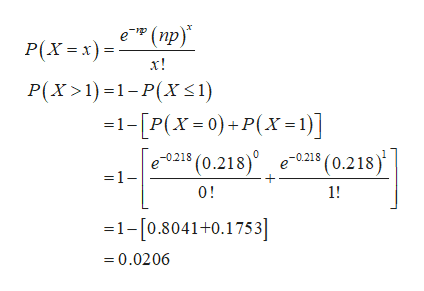# A rare form of malignant tumor occurs in 11 children in a​ million, so its probability is 0.000011. Four cases of this tumor occurred in a certain​ town, which had 19,809 children  d. Let a probability of 0.05 or less be​ "very small," and let a probability of 0.95 or more be​ "very large". Does the cluster of four cases appear to be attributable to random​ chance? Why or why​ not?  A.​Yes, because the probability of more than one case is very large. B.​No, because the probability of more than one case is very large. C.​No, because the probability of more than one case is very small. D.​Yes, because the probability of more than one case is very small.

Question
A rare form of malignant tumor occurs in 11 children in a​ million, so its probability is 0.000011. Four cases of this tumor occurred in a certain​ town, which had 19,809 children

d. Let a probability of 0.05 or less be​ "very small," and let a probability of 0.95 or more be​ "very large". Does the cluster of four cases appear to be attributable to random​ chance? Why or why​ not?

A.
​Yes, because the probability of more than one case is very large.

B.
​No, because the probability of more than one case is very large.

C.
​No, because the probability of more than one case is very small.

D.
​Yes, because the probability of more than one case is very small.
check_circleExpert Solution
Step 1

d.

From the given information, the probability is 0.000011 and the number of children is 19,809. That is, p=0.000011 and n=19,809.

Let us define the random ...help_outlineImage Transcriptionclosee (np) " (пp)" P(xx) х! P(x>1)1-P(X 1) 1-[P(x=0)+P(X=1)] 021 (0.218 -0215 (0.218) =1- 0! 1! -1- [0.8041+0.1753] -0.0206 fullscreen

### Want to see the full answer?

See Solution

#### Want to see this answer and more?

Solutions are written by subject experts who are available 24/7. Questions are typically answered within 1 hour*

See Solution
*Response times may vary by subject and question
Tagged in

### Other